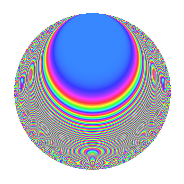# Properties

 Label 215.2.gLevel 215 Weight 2 Character orbit g Rep. character $$\chi_{215}(42,\cdot)$$ Character field $$\Q(\zeta_{4})$$ Dimension 40 Newform subspaces 1 Sturm bound 44 Trace bound 0

# Related objects

## Defining parameters

 Level: $$N$$ = $$215 = 5 \cdot 43$$ Weight: $$k$$ = $$2$$ Character orbit: $$[\chi]$$ = 215.g (of order $$4$$ and degree $$2$$) Character conductor: $$\operatorname{cond}(\chi)$$ = $$215$$ Character field: $$\Q(i)$$ Newform subspaces: $$1$$ Sturm bound: $$44$$ Trace bound: $$0$$

## Dimensions

The following table gives the dimensions of various subspaces of $$M_{2}(215, [\chi])$$.

Total New Old
Modular forms 48 48 0
Cusp forms 40 40 0
Eisenstein series 8 8 0

## Trace form

 $$40q - 16q^{6} + O(q^{10})$$ $$40q - 16q^{6} - 20q^{10} - 8q^{11} + 4q^{13} - 4q^{15} - 20q^{16} - 8q^{17} + 8q^{21} - 28q^{23} - 8q^{25} - 48q^{31} + 24q^{35} + 20q^{36} + 28q^{38} + 68q^{40} + 8q^{41} + 28q^{47} + 8q^{52} + 36q^{53} + 28q^{56} - 36q^{57} + 16q^{58} - 44q^{60} - 52q^{66} - 36q^{67} + 68q^{68} - 132q^{78} + 48q^{81} - 60q^{83} + 12q^{86} - 40q^{87} + 12q^{90} + 168q^{92} + 16q^{95} + 76q^{96} + 20q^{97} + O(q^{100})$$

## Decomposition of $$S_{2}^{\mathrm{new}}(215, [\chi])$$ into newform subspaces

Label Dim. $$A$$ Field CM Traces $q$-expansion
$$a_2$$ $$a_3$$ $$a_5$$ $$a_7$$
215.2.g.a $$40$$ $$1.717$$ None $$0$$ $$0$$ $$0$$ $$0$$

## Hecke Characteristic Polynomials

There are no characteristic polynomials of Hecke operators in the database